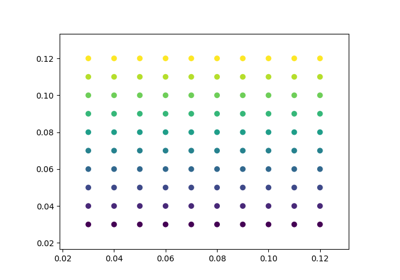# matplotlib.pyplot.rc_context¶

`matplotlib.pyplot.``rc_context`(rc=None, fname=None)[source]

Return a context manager for managing rc settings.

This allows one to do:

```with mpl.rc_context(fname='screen.rc'):
plt.plot(x, a)
with mpl.rc_context(fname='print.rc'):
plt.plot(x, b)
plt.plot(x, c)
```

The ‘a’ vs ‘x’ and ‘c’ vs ‘x’ plots would have settings from ‘screen.rc’, while the ‘b’ vs ‘x’ plot would have settings from ‘print.rc’.

A dictionary can also be passed to the context manager:

```with mpl.rc_context(rc={'text.usetex': True}, fname='screen.rc'):
plt.plot(x, a)
```

The ‘rc’ dictionary takes precedence over the settings loaded from ‘fname’. Passing a dictionary only is also valid. For example a common usage is:

```with mpl.rc_context(rc={'interactive': False}):
fig, ax = plt.subplots()
ax.plot(range(3), range(3))
fig.savefig('A.png', format='png')
plt.close(fig)
```

## Examples using `matplotlib.pyplot.rc_context`¶Automatically setting tick labels